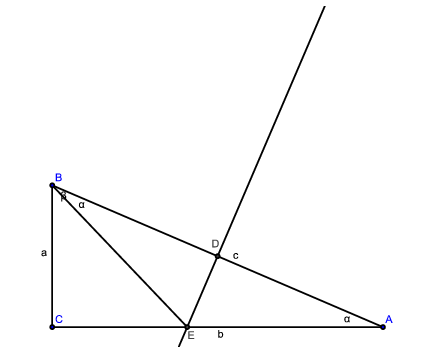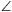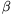Mathematical and Physical Journal
for High Schools
Issued by the MATFUND Foundation
 Already signed up? New to KöMaL?

#Problem C. 919. (November 2007)

C. 919. A right-angled triangle is cut into a triangle and a quadrilateral by the perpendicular bisector of its hypotenuse. The ratio of the diagonals of the quadrilateral is. Find the acute angles of the right-angled triangle.

(5 pont)

Deadline expired on December 17, 2007.

Sorry, the solution is available only in Hungarian. Google translation

Megoldás:Mivel E rajta van az AB szakasz felező merőlegesén, ezért EBA=BAC=., ezért. A Thalesz-tétel miatt BD=DC, így az átlók aránya. Tudjuk, hogy, ezért az átlók aránya:Mivelezért=75o,=15o.

### Statistics:

 274 students sent a solution. 5 points: 232 students. 4 points: 19 students. 3 points: 4 students. 2 points: 4 students. 1 point: 4 students. 0 point: 10 students. Unfair, not evaluated: 1 solutions.

Problems in Mathematics of KöMaL, November 2007